• 问答
• 技术
• 实践
• 资源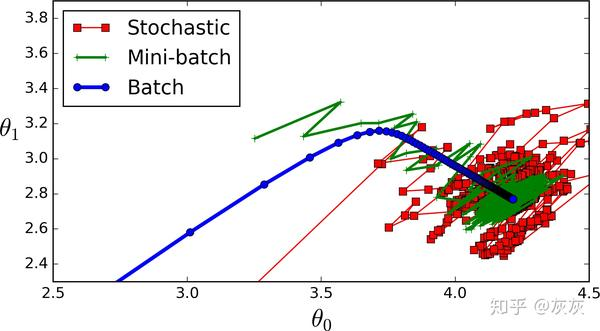1. 简要概述每种算法的作用
2. 算法的代码
3. 对规范不明确部分的进一步解释import numpy as np
import pandas as pd
import plotly.express as px
from sklearn.metrics import mean_squared_error

data = load_boston()

df = pd.DataFrame(data['data'],columns=data['feature_names'])
df.insert(13,'target',data['target'])
df.head(5)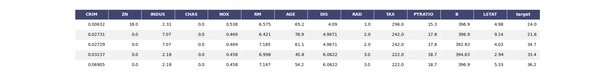X,y = df.drop('target',axis=1),df['target']

thetas = np.zeros(X.shape)

### 成本函数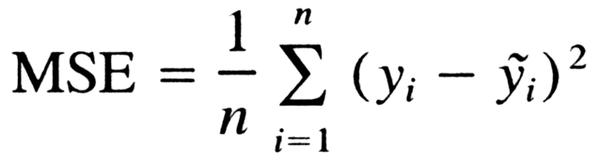def cost_function(X,Y,B):
predictions = np.dot(X,B.T)

cost = (1/len(Y)) * np.sum((predictions - Y) ** 2)
return cost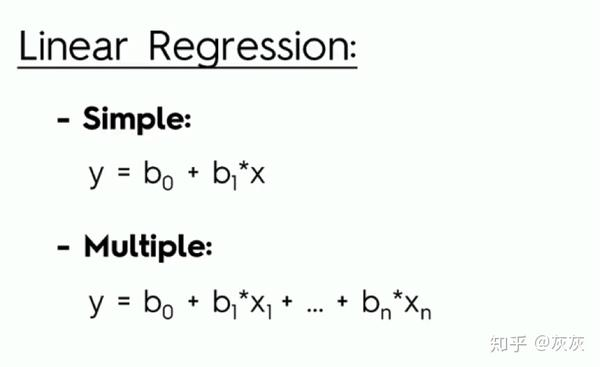mean_squared_error(np.dot(X,thetas.T),y)

OUT: 592.14691169960474

cost_function(X,y,thetas)

OUT: 592.14691169960474

### 特征缩放

1. 你有一座很大的建筑物
2. 你希望保持建筑的形状，但希望将其调整为较小的比例

1. 如果一个算法使用欧几里德距离，那么由于欧几里德距离对较大的量值敏感，因此需要对特征进行缩放
2. 特征缩放还可以用于数据标准化
3. 特征缩放还可以提高算法的速度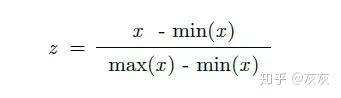X_norm = (X - X.min()) / (X.max() - X.min())
X = X_norm1. 批量梯度下降
2. 随机梯度下降法
3. 小批量梯度下降

### 批量梯度下降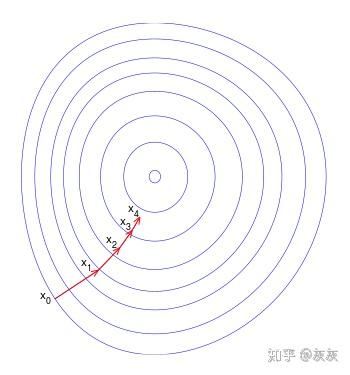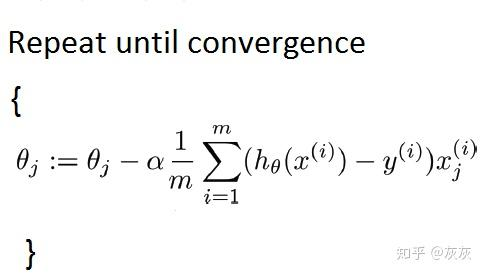def batch_gradient_descent(X,Y,theta,alpha,iters):
cost_history =  * iters  # 初始化历史损失列表
for i in range(iters):
prediction = np.dot(X,theta.T)
theta = theta - (alpha/len(Y)) * np.dot(prediction - Y,X)
cost_history[i] = cost_function(X,Y,theta)
return theta,cost_history

alpha：这是指学习率。

iters：迭代运行的数量。

batch_theta,batch_history=batch_gradient_descent(X,y,theta,0.05,500)

cost_function(X,y,batch_theta)

OUT: 27.537447130784262

fig = px.line(batch_history,x=range(5000),y=batch_history,labels={'x':'no. of iterations','y':'cost function'})
fig.show()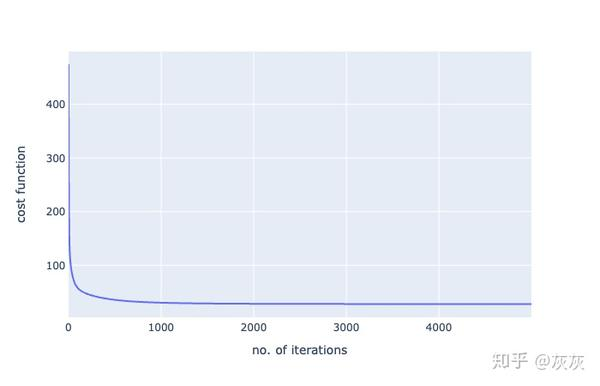### 优点

1. 有效且曲线平滑
2. 最准确，最有可能达到全局最低值

### 缺点

1. 对于大型数据集可能会很慢
2. 计算成本高

### 随机梯度下降法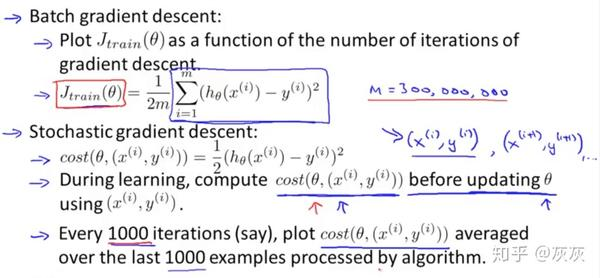### 学习率调整

1. 如果学习速率降低得太快，那么算法可能会陷入局部极小，或者在达到最小值的一半时停滞不前；
2. 如果学习速率降低太慢，可能会在很长一段时间内跳转到最小值附近，仍然无法得到最佳参数。

t0,t1 = 5,50 # 学习率超参数

def learning_schedule(t):
return t0/(t+t1)

c_hist =  * n_epochs # 历史成本
for epoch in range(n_epochs):
for i in range(len(y)):
random_index = np.random.randint(len(Y))
xi = X[random_index:random_index+1]
yi = y[random_index:random_index+1]

prediction = xi.dot(thetas)

eta = learning_schedule(epoch * len(Y) + i)
thetas = thetas - eta * gradient
c_hist[epoch] = cost_function(xi,yi,thetas)
return thetas,c_hist

sdg_thetas,sgd_cost_hist = stochastic_gradient_descent(X,Y,theta)

cost_function(X,y,sdg_thetas)

OUT:
29.833230764634493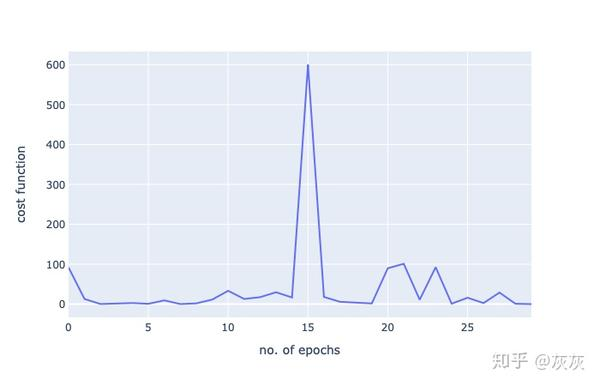1. 与批量梯度下降相比更快
2. 更好地处理更大的数据集

### 缺点：

1. 在某个最小值上很难跳出
2. 并不总是有一个清晰的图，可以在一个最小值附近反弹，但永远不会达到最佳的最小值

### 小批量梯度下降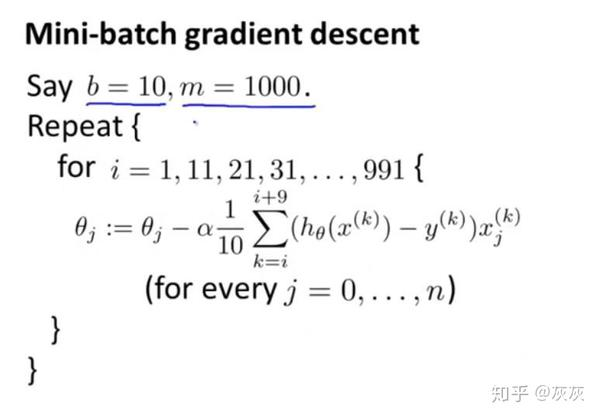np.random.seed(42) # 所以我们得到相同的结果

t0, t1 = 200, 1000
def learning_schedule(t):
return t0 / (t + t1)

t = 0
c_hist =  * n_iters
for epoch in range(n_iters):
shuffled_indices = np.random.permutation(len(y))
X_shuffled = X_scaled[shuffled_indices]
y_shuffled = y[shuffled_indices]

for i in range(0,len(Y),batch_size):
t+=1
xi = X_shuffled[i:i+batch_size]
yi = y_shuffled[i:i+batch_size]

gradient = 2/batch_size * xi.T.dot(xi.dot(thetas) - yi)
eta = learning_schedule(t)
thetas = thetas - eta * gradient
c_hist[epoch] = cost_function(xi,yi,thetas)
return thetas,c_hist

mini_batch_gd_thetas,mini_batch_gd_cost = mini_batch_gradient_descent(X,y,theta)

cost_function(X,Y,mini_batch_gd_thetas)

OUT: 27.509689139167012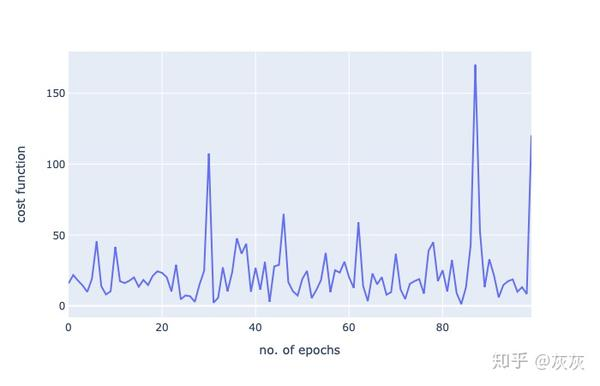•0
•0
•1687收藏

• 1

关注
• 1

获赞
• 2

精选文章

• 18296160229的专栏

• 3.0k
• 1.9k
• 2.2k
• 2.3k
• 1.6k